# 2nd PUC Basic Maths Question Bank Chapter 3 Probability Ex 3.1

Students can Download Basic Maths Exercise 3.1 Questions and Answers, Notes Pdf, 2nd PUC Basic Maths Question Bank with Answers helps you to revise the complete Karnataka State Board Syllabus and score more marks in your examinations.

## Karnataka 2nd PUC Basic Maths Question Bank Chapter 3 Probability Ex 3.1

Part – A

2nd PUC Basic Maths Probability Ex 3.1 One Mark Questions and Answers

Question 1.
What is an Experiment?
An operation which can produce some well defined outcomes in known as an experiment.

Question 2.
What is random experiment?
Any experiment that results in two to more outcomes is called a random experiment.

Question 3.
Define sample space.
The set of all possible outcomes of a random experiment is called as sample space & it is denoted by S.

Question 4.
What is the probability of sample space?
P(S) = 1Question 5.
What is an event?
Any subset of a sample space is called an event.

Question 6.
What is the range for probability?
Range of probability is 0 ≤ P(A) ≤ 1.

Question 7.
What is probability of a certain event?
p(certain event) = 1

Question 8.
What is probability of an impossible event?
P(Impossible event) = 0

Question 9.
Define
(aj Mutually exclusive events
(b) Independent events
(c) Complementary events.
(a) If two events cannot occur simultaneously in a random experiment then they are called mutually exclusive events,
(b) Events are said to be independent, if the occurrence of one does not depend upon the occurrence of the other,
(c) In a random experiment, let S be the sample space & let E be an event then E C S, So ‘E’ is also an event called the complementary of E.

Question 10.
IfP(A) = $$\frac{3}{5}$$ FindP(A’).
If P(A) = $$\frac{3}{5}$$ then P(A’) = 1-P(A) = 1- $$\frac{3}{5}$$ = $$\frac{2}{5}$$Question 11.
A coin is tossed thrice. Write the sample space.
S = (HHH, HHT, HTH, HTT, THH, TTH, THT, TTT}

Question 12.
Ved hits the target 7 times out of 10 shots. Find his probability of missing the target.
P[Missing the target = $$1-\frac{7}{10}=\frac{10-7}{10}=\frac{3}{10}$$

Question 13.
What is the probability of an event A if odds in favour are 3:5.
P(odds in favour of 3 : 5) = $$\frac{3}{8}$$

Question 14.
What is the probability of an event A if odds against are 2:7
(odds against of 2 : 7) = $$\frac{7}{9}$$

Question 15.
A card is drawn from a pack of 52 playing cards What is the probability that is queen.
Event A: Card is a queen => n(A) = 4 & n(S) = 52.
P(A) = $$\frac{n(A)}{n(S)}=\frac{4}{52}$$

Question 16.
What is the probability of getting two heads when a coin is tossed twice.
S = {HH, 1IT, TH, IT}, n(S) = 4
Event A = Two heads n(A) = 1.
∴ P(A) = $$\frac{n(A)}{n(S)}=\frac{1}{4}$$

Question 17.
If P(A) = $$\frac{3}{4}$$ ,P(B) = $$\frac{1}{2}$$ PAB) = $$\frac{1}{4}$$ , fine P(A/B)Question 18.
A bag contains 8 red and 4 green marbles. Find the probability that a marble selected at random is red.
n(s) = 8 + 4 = 12
A: Marble is red, n(A) = 8
P(A) = $$\frac{n(A)}{n(S)}=\frac{8}{12}=\frac{2}{3}$$

Question 19.
What is the probability of getting a multiple of 3 when a die is thrown.
n(S) = 6, A = {3,6}, n(A = 2
∴ P(A) = $$\frac{n(A)}{n(S)}=\frac{2}{6}=\frac{1}{3}$$
n(S) 6 3

Question 20.
What is the probability that the child born is a boy?
S = {B,G} n(S) = 2.
A: Child is a boy, n(A) = 1
P(A) = $$\frac{\mathrm{n}(\mathrm{A})}{\mathrm{n}(\mathrm{S})}=\frac{1}{2}$$

Question 21.
A number chosen from 1 to 50. What is the probability that the number is a multiple of 8.
n(S) = 50, A = {8, 16, 24, 32, 40, 48}
n(A)=6
P(A) = $$\frac{6}{50}=\frac{3}{25}$$

Question 22.
If A and B are mutually exhaustive events, then what is P(A ∪ B)
P (A ∪ B) = P(A) + P(B) – P (A∩B)Question 23.
A die is thrown. What is the chance of getting a face with dots as multiple of 2.
n(S) = 6,A = {24,6}
P(A) = $$\frac{n(A)}{n(S)}=\frac{3}{6}=\frac{1}{2}$$

Question 24.
If A and B are independent events find P(B/A)
If A and B are independent events P(A ∩ B) = P(A). P(B)Question 25.
A box contains 5red balls, 8 green balls and 10 pink balls. A ball is drawn at random from the box. What
is the probability that the ball drawn is either red or green.
m(S) = 5 + 8 + 10 = 23
A= Red orGreen, nÍA)= 5+8=13
P(A) = $$\frac{n(A)}{n(S)}=\frac{13}{23}$$

Part – B

2nd PUC Basic Maths Probability Ex 3.1 Two Mark Questions and Answers

Question 1.
What are independent events? Give one example,
(a) If two events cannot occur simultaneously in a random experiment then they are called mutually exclusive events
(b) Events are said to be independent, if the occurrence of one does not depend upon the occurrence of the other
(c) In a random experiment, let S be the sample space & let E be an event then E C S, So ‘E’ is also an event called the complementary of E.

Question 2.
Explain sample space with an example.
The set of all possible outcomes of a random experiment is called as sample space & it is denoted by S.
S = (HHH, HHT, HTH, HTT, THH, TTH, THT, TTT}

Question 3.
IfA and B are mutually exclusive events with P(A) = $$\frac{2}{5}$$ P(B) = $$\frac{1}{7}$$ find P(A ∪ B)
P(A ∪ B) = P(A) + P(B)∵A & B are mutually exclusive.

Question 4.
If P(A) = $$\frac{1}{2}$$ P(B) = $$\frac{1}{3}$$ P(A∪B} = $$\frac{7}{12}$$ find P(B/A)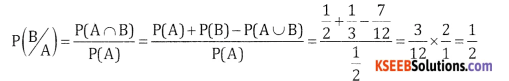Question 5.
If P(A’) = 0.65 P(A∪B) = 0.65 find P(B)
If P(A’) = 0.65 ⇒ P(A) = 1 – 0.65 = 0.35
P(A∪B) = P(A)+P(B)
0.65 = 0.35 + P(B) ⇒ P(B) = 0.65 – 0.35 = 0.30Question 6.
Two cards are drawn at random from a well-shuffled pack of 52 cards. Wh both are queens or both are king cards,
Here n(S) = 52c2 = $$\frac{52 \times 51}{2 \times 1}$$ = 1326
Let Event A: both are queeWs, n(A)=4c2 = $$\frac{4 \times 3}{2 \times 1}$$ =6
Event B: both are kings, n(B) = 4c2 = $$\frac{4 \times 3}{2 \times 1}$$ = 6
A & B are mutually exclusive A ∩ B = OQuestion 7.
A die is thrown twice, what is the probability that at ¡east one of the two numbers is 6.
n(S) = = 36
Event A = {(1, 6), (2, 6) (3, 6) (4, 6) (5, 6) (6,6) (6, 1) (6, 2) (6, 3) (6, 4) (6, 5)}
n(A) = 11
∴ P(A) = $$\frac{\mathrm{n}(\mathrm{A})}{\mathrm{n}(\mathrm{S})}=\frac{11}{36}$$

Question 8.
From a well-shuffled pack of 52 cards a card is drawn at random. Find probability that the drawn card is a king or a queen.
n(S) = 52
Event A:Cardisakingz ⇒ n(A)=4
B = Card is a Queen ⇒ n(B) = 4
A & B are mutually exclusive (A ∩ B) = (A ∩ B) = 9, P(A or B) = P(A∪B) = P(A) +P(B)Question 9.
The probability of occurrence of two events A and B are $$\frac{1}{4}$$ and $$\frac{1}{2}$$ respectively. The probability of their simultaneous occurrence is $$\frac{7}{50}$$ . What is the probability that neither A nor B occurs.
[Hint:find 1- P(A∪3)
‘Given P(A) = $$\frac{1}{4}$$, P(B) = $$\frac{1}{2}$$
P(A∩ B) = $$\frac{7}{5}$$
P(neither A nor B) = P(A’∩B’) = P(A∪B)’ = 1 – P(A∪B)
=1 – [P(A)+P(B)-P(A∩B)]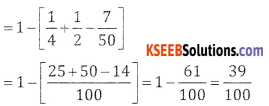Question 10.
Two coins are tossed simultaneously. What is the probability of getting (a) atmost 1 tail (b) atleast 1 tail.
(a) S = {HH, HT, TH, TT}, n(S) = 4
Let A: Atmost 1 tail
A = {HH, HT, TH}, n(A) = 3, P(A) = $$\frac{3}{4}$$
(b) B = Atleastl tail
B = {HT,TH,TT}, n(B) = 3.P(B) = $$\frac{3}{4}$$

Question 11.
Three fair coins are tossed simultaneously. Find the probability of getting atleast one head and aleast one tail.
S = {HHH, HHT, HTH, THH, HTT, THT, TTH, TTT}
n(S)=8
Let A: Atleast one head & atleast onetail.
A = {HTH, HHT, HTT, THH, THT, TTH}, n(A) =6
P(A) = $$\frac{n(A)}{n(S)}=\frac{6}{8}=\frac{3}{4}$$

Question 12.
Two dice are rolled simultaneously. Find the probability of getting a doublet of even numbers.
n(S) = 36
Let A=doublet of even numbers
A = {(2, 2), (4, 4), (6, 6)), n(A) = 3
P(A) = $$\frac{n(A)}{n(S)}=\frac{3}{36}=\frac{1}{12}$$

Question 13.
If there are two children in a family. Find the probability that there ¡s atleast one girl child in the family.
Li S={HB,BG,GB,GG),n(S)=4
A: Atleast one girl,
A={BG,GB,GG},n(A)=3
P(A) = $$\frac{\mathrm{n}(\mathrm{A})}{\mathrm{n}(\mathrm{S})}=\frac{3}{4}$$

Question 14.
A box contains S defective and 15 non defective bulbs. Two bulbs are chosen at random. Find the probability that both the bulbs are non- defective.
Total = 5 + 15 = 20,
n(S)= 20c2 = $$\frac{20 \times 19}{2 \times 1}$$= 190
Let A = both bulbs are non-defective
n(A)= 15c2= $$\frac{14 \times 15}{2 \times 1}$$ = 105
P(A)= $$\frac{n(A)}{n(S)}=\frac{105}{190}=\frac{21}{38}$$Question 15.
A problem in a question paper is given to 3 students in a class to be solved. The probabilities of their solving the problem are 0.5, 0.7 and 0.8 respectively. Find the probability that the problem will be solved.
Let
P(A) = P(A Solves the problem) = 0.5
P(B) = P(B solves the problem) = 0.7
P(C) = P(C solves the problem) = 0.8
P(Problem is solved)
= 1 – P(None of then solves O
= 1 – P(A’ B’ C’) = 1 – F(A’) F(B’) P(C’)
=1 – (0.5)(0.3)(0.2) = 1 – 0.03 = 0.97

Question 16.
What is the probability that a randomly chosen two-digit positive integer is a multiple of 3.
Let n(s) 90
A={12,15 ……………… 99}
n(A)=30
P(A) = $$\frac{n(A)}{n(S)}=\frac{30}{90}=\frac{1}{3}$$
Using a + (n — 1)d = Tn
12+ (n – 1)3=99
12 + 3x – 3 = 99
3x = 99 – 9
3x = 90; x = 30

Question 17.
Two cards are drawn from a pack of 52 cards. What is the probability that both are face cards.
n(s) = 52c2 = $$\frac{52 \times 51}{2 \times 1}$$ = 1326
Let A = both are face cards
n(s) = 12c2 = $$\frac{12 \times 11}{2 \times 1}$$ = 66
n(s) = 52c2 = $$\frac{52 \times 51}{2 \times 1}$$ = 1326
P(A) = $$\frac{n(A)}{n(S)}=\frac{66}{1326}=\frac{11}{221}$$

Question 18.
Tickets are numbered from 1 to 18 are mixed up together and one ticket is drawn at random. What is the probability that the ticket has a number which is a multiple of 2 or 3.
n(S) = 18
Let A: Multiple of 2, A {2 ………………..18), n(A) = 9
B: Multiple of 3, B {3, …………… 18), n(B) = 6
A ∩ B: Multiple of 2 & 3, A B {6, 12, 18}, n(A ∩ B) = 3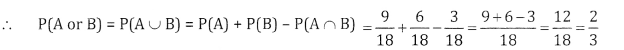Question 19.
If the letters of the word ‘RAMLEELA’ are arranged in random. What is the probability that it begins with REEL.
n(s) = $$\frac{8 !}{(21)^{3}}$$
Let A: Word begins with REEL
n(A) = $$\frac{4 !}{2 !}$$Question 20.
A committee of 4 has to be selected from 9 boys and 6 girls. What is the probability that the corn contains 2 boys and 2 girls.
n(S) (9 + 6)C4 =15c4
Let A: Committees contains 2 Boys and 2 Girls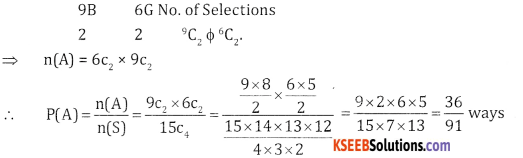Part – C

2nd PUC Basic Maths Probability Ex 3.1 Three Marks Questions and Answers

Question 1.
A box contains 4 defective and 6 non def when 4 bulbs are selected at random.
n(S) = (6 + 4)C4 = 10 $$c_{4}-\frac{10 \times 9 \times 8 \times 7}{4 \times 3 \times 2 \times 1}$$ = 210
Let A: at least 3 are defective
Here 4 defective 6non defectiveQuestion 2.
A natural number is chosen at random from among the first 300. What Is the probability that the number
so chosen is divisible by 3 or 5.
n(S) = 300
Let A: Number is divisible by 3 = (3, 6, 9 300)
∴ n(A) = 100
Let B: Number is divisible by 5 = (5, 10 300)
∴ n(B) = 60
A∩ B: Number is divisible by 3 & 5 i.e., 15
A∩B = {15 ………………….300} n(A∩B) = $$\frac{300}{15}$$ = 20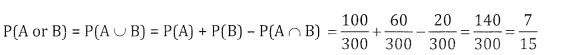Question 3.
The probability that a MBA aspirant will join 11M is $$\frac{2}{5}$$ and that he will join XLRI is $$\frac{1}{3}$$ . Find the probability that (a.) he will join 11M or XLRI
(b) he will join neither 11M nor XLRI.
‘Let A: MBA aspirant will join TIM ⇒ P(A) = $$\frac{2}{5}$$
B: Will join XLRI ⇒ P(B) = $$\frac{1}{3}$$
A ∩ B = 9
∴ P(JoinhIMor x LRl) = P(A∪ B) = P(A) + (PB) = $$\frac{2}{5}+\frac{1}{3}=\frac{11}{15}$$
P(NeitherllM norx LRI)= P(A’∪ B’) = P(A∪ B)’ = 1 – P(A∪B)
= $$1-\frac{11}{15}=\frac{15-11}{15}=\frac{4}{15}$$

Question 4.
A die is thrown twice and sum of the numbers appearing is observed to be 9. What is the conditional probability that the number 4 has appeared at least once.
Let A:Sum is 9
A = {(3,6) (6, 3) (5,4) (4,5)} ⇒ n(A)=4
B = (1, 4) (2, 4) (3, 4) (4, 4) (5, 4) (6, 4) (6, 4) (4, 1) (4, 2) (4, 3) (4, 5) (4, 6)
A∩B = {(5,4)(4,5)} ⇒ n(A∩B)=2Question 5.
A die is rolled. If the outcome is an odd number. What Is the probability that It is a number greater than 1.
Let A: Odd number = {1, 3, 5) ⇒ n(A) = 3
B: number greater than 1 = {2, 3, 4, 5, 6)} ⇒ n(S) = 5
A∩B ={3,5} ⇒ n(A∩B) = 2Question 6.
A couple has two children. Find the probability that both are boys, ¡fit is known that
(a) one of the children is a boy
(b) older child is a boy.
(a) Let
A: both are boys={B, B}, n(A)= 1
Let B: One of the child is boy = {BG, BB, GB}
= n(B) = 3
(b) Let C: Elder child is a boy
c={BG,BB} ⇒ n(c) = $$\frac{2}{4}$$Question 7.
A die is rolled twice. If the sum of the numbers facing upwards is even find the probability that both are odd
Let A: both are odd
A = {(1, 1) (1, 3) (1, 5) (3, 1) (3, 3) (3, 5) (5. 3) (5, 1) (5, 5))}
n(A)=9
B = sum is even
= {((1, 1) (1, 3) (1, 5) (3, 1) (3, 3) (3, 5) (5, 3) (5, 1) (5, 5) (2, 2) (2, 4) (2, 6) (4, 2) (4, 4) (4, 6) (6, 2) (6. 4) (6, 6))}
n(B) 18
n(A∩B)=9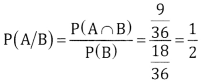Question 8.
Seven persons are to be seated in a row. Find the probability that 2 particular persons sit next to each other.
Here n(S) = 7!
Let A: 2 persons sit next to each other.
Let the 2 particular persons be together and 5 remaining people, totally 6 can be permuted in 6! ways and 2 particular persons they themselves can be permuted in 2! Ways.
∴ the number of ways is 6! And 2!.Question 9.
There are 20 girls and 60 boys in a class. Half of the girls and half of the boys are first class students. A student ¡s selected at random. What is the probability that the student ¡s either a boy or a first class holder.
n(S) = 20 + 60 = 80
Let A: student is a boy n(A) 60
P(A) = $$\frac{60}{80}=\frac{3}{4}$$
Let B: student is a ¡ class holder
1 class = 30 boys + lo girls = 40
P(B) = $$\frac{40}{80}=\frac{1}{2}$$
Let (A ∩ B) = n(Student is a boy and I class holder) = 30
P(A) = (A ∩ B) = $$\frac{30}{80}=\frac{3}{8}$$
∴ P(Student is a boy of first class holder)Question 10.
Among the members of a committee, there are 75% males and 25% females. The probability that a male member becomes the president is 0.25 and probability that a female member becomes the president is 0.4. Find the probability that the person selected at random becomes the president.
[Hint: Person selected Is Male and becomes President OR is female and becomes President]
Let A:Malemember = P(A) = $$\frac{75}{100}=\frac{3}{4}$$
B: Female member =P(B) = $$\frac{25}{100}=\frac{1}{4}$$
C: Member is a president
P(C/A) = 0 25, P(Female president) = P(C/B) = 0.4
P(C) = P(Member is a president)
= P(A) P(C/A) + P(B)P(C/B)Question 11.
Two cards are drawn from a pack of playing cards, one after the other. Find the probability of getting a
heart in first draw and diamond ¡n the second draw if the cards are drawn.
(a) without replacement
(b) with replacement
(a) Without replacement
Heart in I draw and claimant in II draw
∴ n(A)= 13C1 x 13C1 n(S)= 52C1 x 51C1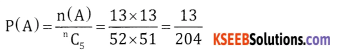(b) With replacement
n(S) = 52 x 52 and n(A) = 13 x 13Question 12.
If three cards are drawn at random from a pack of 52 cards, what is the probability that at least two of them are kIngs?Part-D

2nd PUC Basic Maths Probability Ex 3.1 Five Marks Questions and Answers

Question 1.
If the letters of the Word INDEPENDENCEare arranged at random. Find the probability that
(a) 4 E’s are together in the word.
(b) The 2 D’s are together and 3 N’s are togethc
(c) All the 4 E’s are not together.
(d) No two E’s are together.
Total letters = 12 in which N = 3, D = 2. E = 4.
Total number of Arrangements = n(S) = $$\frac{12 !}{3 ! \cdot 2 ! \cdot 4 !}$$

(a) A : 4 E’s are together can be taken as I unit and remaining 8 totally 9 can be permuted in
$$\frac{9 !}{3 ! \cdot 2 !}$$(b) B: 2D’s are together and 3N’s are together
1 + 1 + remaining 7 = 9 can be done in $$\frac{9 !}{4 !}$$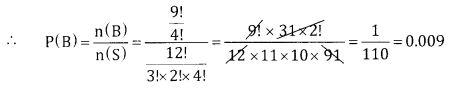(c) All 4E’s are not together = total – together = 1 – 0.0 18 = 0.982
(d) D: No. two Es are together
:. E’s are in between the other B letters, there are 9 spaces, in which 4 Es are placed in 9C4 ways and 8 letters can be permuted in $$\frac{8 !}{3 ! \times 2 !}$$ and 4E’s are repeated need to delete n(D) = $$^{9} \mathrm{C}_{4} \frac{8 !}{3 ! \cdot 2 ! \times 4 !}$$Question 2.
A bag contains 3 red and 4 black balls and another bag has 4 red and 2 black halls. One bag is selected at
random and from the selected bag a ball Is drawn. Let E be the event that the flrstbag ¡s séÍected, F b the
event that the second bag is selected. G be the event that ball drawn Is red. Find
(a) P(E)
(b) P(F)
(c) P(G/E)
(d) P(G/F)
Let E: First bag is selected
(a) P(E) = $$\frac{1}{2}$$
F: Second bag is selected G: Ball is red
(b) P(F) = $$\frac{1}{2}$$
G: Ball is Red
(c) P (G/E) = P (red ball from I bag) = $$\frac{3}{4}$$
(d) P(G/F)= P(red ball from Il bag) = $$\frac{4}{6}=\frac{2}{3}$$

Question 3.
In a class 45% students read English, 30% read French and 20% read both English and trench. one student Is selected at random. Find the probability that a) he reads english, If It Is known that he reads French b) he reads French [f It Is known that he reads english
E: 45% red English = P(E) = $$\frac{45}{100}$$
F: 30% red French P(F) = $$\frac{30}{100}$$
E m F: 20% read both English & French P(E ∩ F) = $$\frac{200}{100}$$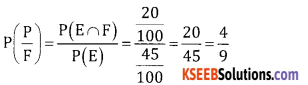Question 4.
A bag contains 8 red and 4 green balls. Find the probability th
(a) ball drawn is red when one ball is selected at random.
(b) all the 4 baUs are green when four balls are drawn at random.
(c) Two balls are red and one ball is green when three balls are drawn at r
(d) Three balls are drawn and none of them Is green.
n(s)=8+4= 12Question 5.
In case of 1oo students, 60 drInk tea, 50 drInk coffee and 30 think both tea and coffee A chidgini fvva this class is selected at random. What Is the probability that the student takes
(a) atleast one õf the two drinks
(b) only one of the drinks
Let E: Students drinks tea ⇒ P(E) = $$\frac{60}{100}=\frac{3}{5}$$
F: Student drinks coffee ⇒ P(F) = $$\frac{50}{100}=\frac{1}{2}$$
E n F: Student drinks both tea & coffee P (E ∩ F) = $$\frac{30}{100}=\frac{3}{10}$$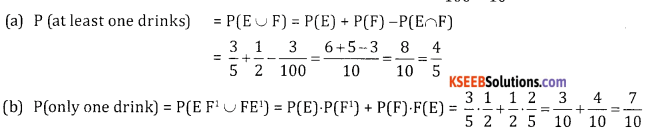Question 6.
One card is drawn from a oi L ciras. rina iiae pruuauuiry war
(a) Card is neither an ace nor a king
(b) Queen given the
(c) Card is either black or a jack
(d) It Is a face card
n(s) = 52, E = Ace, F = king
(a) Let A: card is neither ace nor a king E’ ∩ F’
P(E) = $$\frac{4}{52}$$ P(F) = $$\frac{4}{52}$$ P(E∩ F) = 0
P(A) = P(E’ ∩ F’) = P(E∪ F)’ = 1 – P(E∪F)(b) B: Queen given that the card is red
Let R: Card is queen P P(R) = $$\frac{4}{52}[latex] S: Card is red P P(S) = [latex]\frac{26}{52}=\frac{1}{2}$$
R m S: Card is red & Queen P P(R∩S) = $$\frac{2}{52}$$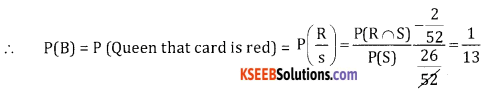(c) Let C: Card is black or a jack LUM
Where L: Card is black, m : card is jack(e) Let D: Face card (there are 12 face cards)
P(d) = $$\frac{12}{52}=\frac{3}{13}$$

Question 7.
Two dice are rolled simultaneously. Find the probability of
(a) getting a total of 11.
(b) getting a sum greater thar 11
(c) getting a multiple of 2 on one die and a multiple of 3 on the other.
N(S) = 36
Let A : sum is 11 = {(6, 5) (5, 6)) ⇒ n(A) = 2
(a) P(A) = $$\frac{n(A)}{n(s)}=\frac{2}{36}=\frac{1}{18}$$

(b) Let B=Sum> 11 = {(66)),n(B) = 1
P(B) = $$\frac{n(B)}{b(s)}=\frac{1}{36}$$
(c) Let C = multiple of 2 on one die & multiple of 3 on the other {(2, 3), (2, 6), (4, 3) (4, 6) (6, 3) (6, 6)} n(c) =
6 P(c) = $$\frac{6}{36}=\frac{1}{6}$$
S = (HHH, HHT, HTH, THIL TTH, THT, HTT, TTT}

Question 8.
Three fair coins are tossed simuItaneousIy Find the proaint
(b) getti
(d) getting 3 heads when It Is known that first two are heads.
n(S) = 8
(a) A: getting one Head = (HTH, HTT, TTH) = n(A) = 3 P(A)= $$\frac{3}{8}$$
(b) B : getting atmost one head (TTT TTH, ThT, HTT) n(B) = 4 P(B) = $$\frac{4}{8}=\frac{1}{2}$$
(c) C : getting atleast two heads = {HHT, THH, FITH, HFŠH}, n(C) = $$\frac{4}{8}=\frac{1}{2}$$
(d) D: first 2 are heads, E: 3 heads $$P\left(\frac{E}{D}\right)=\frac{P(E \cap D)}{P(D)}=\frac{1}{2}$$

Question 9.
Two cards are drawn at random from a pack of cards, Find the probability that both are aces if the
cards are drawn I) together II) one after the other without replacement III) one after the other with
replacement.
n(S) = 52C2
(i) Let A both are ace ⇒ n(A) = 4C2(ii) One after the other without replacement
n(s) = 52 x 51,n(A) = 4 x 3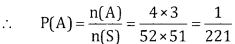(iii) with replacement
n(s) = 52 x 52,n(A) = 4 x 4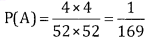Question 10.
A committee of 12 with atleast 5 women has to formed from 9 women and 8 men. What Is the probability that
(a) Women are in majority
(b) Men are in majority In the committee so formed.
n(S) = (9+8)C12 = 6186
(a) Let A : women are in majority
No of selections are: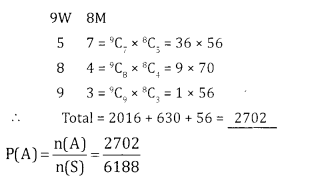(b) Men are in majority
No of selections are: 9w 8M 5 79Cr5 x 8C7, = 126 x 8 = 1008
P(B) = $$\frac{n(B)}{n(S)}=\frac{1008}{6188}$$

Question 11.
From 8 gentlemen and 7 ladies a committee of 5 Is to be formed. What is the probability that this committee consists of
(b) atleast 3 gentlemen .
n(S) = (8+7)C5 = 15C5 = 3003
(a)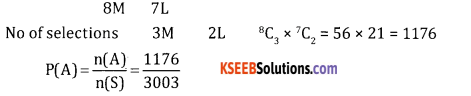(b) Let B : At least 3 gentlemen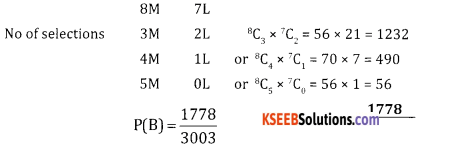(c) Let C : At most 2 LadiesQuestion 12.
There are six tickets numbered from O to 5. Two tickets are selected at random from the lot. What is the probability that the sum Is
(a) atmost 8
(b) atleast 8
(c) prime number
n(S) = 6C2 = $$\frac{6 \times 5}{2 \times 1}$$ = 15
(a) Let A: sum is at most 8 i.e max B
A = {(O, 1) (0, 2) (0,3) (0,4) (0,5) (1,2) (1,3) (1.4) (1,5) (2,3) (2, 4) (2, 5) (3, 4) (3, 5))
n(A)=14
P(A) = $$\frac{n(A)}{n(S)}=\frac{14}{15}$$
(b) Let B = sum is at least 8 = {(5, 3) (4, 5)) ⇒ n(B) = 2
⇒ n(B) = $$\frac{n(B)}{n(S)}=\frac{2}{15}$$
(c) Let C : Sum is prime number
C = { (0. 1) (0, 2) (0, 3) (0, 5) (1, 2) (1. 4) (2, 3) (3, 4)) ⇒ n(C) = 8
P(C) = $$\frac{\mathrm{n}(\mathrm{C})}{\mathrm{n}(\mathrm{S})}=\frac{8}{15}$$

Question 13.
A bag contains 6 red, 4 white and Z black balls. Z balls are drawn at random. What is the probability that the balls drawn are
(a) both red
(b) 1 white and 1 black
(c) same colour
(d) different colour
Here n(s) = (6 + 4. 2)C, = 12C,
(a) Let A:both are red ⇒ n(A)=6C2(b) Let B: 1 white & 1 Black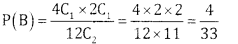(c) Let C1: Both are of same colour.
n(c) = 64c2 or 2Ca = 15 + 6 + 1 = 22(d) Let D both are of different colour no of selections 6C1 x 4C1 or 4C1 x 2C or 6C1 x 2C1 = 24 + 8 + 12 = 44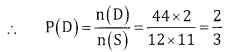Question 14.
An urn contain 4white 2 green and 4 black balls. 3 balls are drawn at random. What is the probability that the drawn balls are 1) 3 white 2) 1 white and 2 green 3) one of each colour.
n(S)=(4+2+4)C3 = 10C3 = $$\frac{10 \times 9 \times 8}{3 \times 2 \times 1}$$ = 120
(1) Let A = 3 white balls ⇒ n(A) = 4C3 = 1
P(A) = =$$\frac{\mathrm{n}(\mathrm{A})}{\mathrm{n}(\mathrm{A})}=\frac{1}{120}$$

(2) Let B: 1 white or 2 green
n(B) = 4C1 x 2C2 = 4 x 1= 4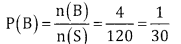(3) Let C1: one of each colour:
n(B) = 4C1 x 2C1 x 4C1 = 4x2 x = 32.Question 15.
The probability that Anirudh solves the given problem is ½and probability that Akansh solves the given problem is $$\frac{1}{4}$$. If the problem Is independently tried by them. What is the probability that
(a) the problem is solved
(b) both of them solve the problem
(c) Anirudh alone solves the problem
(d) Akansh alone solves the problem
(e) Both do not solve the problemQuestion 16.
An educational institute wants to select 3 teachers. If S ladies and 4 gents appear for the interview. What is the probability that the selected teachers are a) 3 gents b) 3 ladies C) 2 gents and I lady d) atleast one lady.

n(s) = (5+4)C3 = 9C3 = $$\frac{9 \times 8 \times 7}{3 \times 2}$$ = 84
(a)Let A:3gents = n(A) = 4C3= 4C1 = 4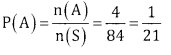(b) Let B : 3 ladies n(B) = 5C3 = $$\frac{5 \times 4 \times 3}{3 \times 2}$$ = 10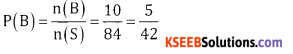(c) Let C:2gents& 1 lady n(C) = 4C2 x 5C1(d) Let D: At least one lady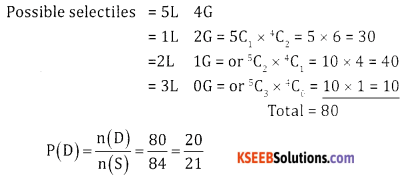Question 17.
Two persons A and B climb a hill. The probability that A climbs the hill is $$\frac{1}{6}$$ and that B climbs the hill is $$\frac{1}{4}$$ . What Is the probability that
(a) Both of them climb the hill
(b) Only one of them will climb the hill
(c) None of them will climb the hill
(d) Only A will climb the hill
(e) Only B climbs the hill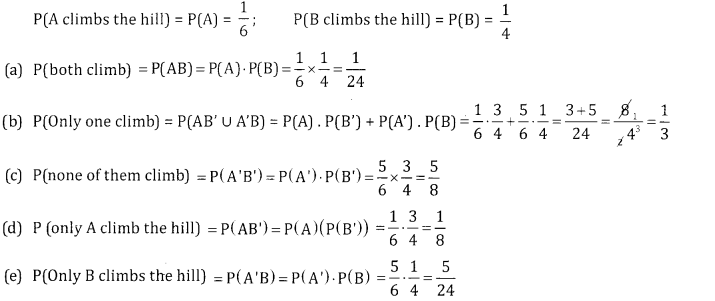Question 18.
The probability that India win a cricket test against Australia is $$\frac{1}{3}$$. If india and Australia nIai
Is the probability that 3
(a) India will lose alt the 3 matches.
(b) India will win all the 3 matches.
(c) India will atleast one match.
(d) India will win exactly two matches.
Given P(India winds) = P(W) = $$\frac{1}{3}$$
P(lndia loses) = P(L) = P(W’) – 1- $$\frac{1}{3}$$ = $$\frac{2}{3}$$
(a) P(lndia loses all 3 matches) P(L.L.L) = P(L) P(L) P(L) = $$\left(\frac{2}{3}\right)^{3}=\frac{8}{27}$$
(b) P(India will all 3 matches) P(WWW) = P( W ) P(W). P(W) = $$\left(\frac{1}{3}\right)^{3}=\frac{1}{27}$$
(c) P(lndia wins atleast one match)
= P(wins 1 match or 2 matchs or 3 matchs)
= 3P(WLL) 3P(WWL) + P(WWW) = 3P(W). P(L) . P(L) + 3P(W) . P(W) . P(L) + P(W) . P(W) . P(W)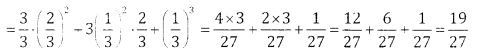(d) P(lndia win exactly 2 matches)
= 3p(\Y\rL) + P(WLW) + P(L\VW)= 3P(WWL)
= 3. P(W). P(Wj. P(L) = $$\left.3 \cdot \frac{1}{3}\right)^{2} \cdot \frac{2}{3}=\frac{2}{9}$$Question 19.
A couple appears in an interview for two vacancies in the same post. The probability of husband’s
selection $$\frac{1}{7}$$ and the probability of wife’s selection is $$\frac{1}{5}$$. What is the probability that
(a) both of them are selected
(b) only one of them will be selected
(c) none of them will be selected
(d) Wife only ¡s selected.
Given P(Husband is selected) = P H) = $$\frac{1}{7}$$
P(H’) = 1 – $$\frac{1}{7}$$ = $$\frac{6}{7}$$
P(wife is selected) = P(W) = $$\frac{1}{5}$$ P(W’) = $$1-\frac{1}{5}=\frac{4}{5}$$

(a) P(both are selected) = P(HW) = P(H).P(W) = $$\frac{1}{7} \cdot \frac{1}{5}=\frac{1}{35}$$
(b) P(only one of them will be selected)
=P(HWHW)=P(H).P(W1)+ P(F1’)P(W)(c) P(None of them will be selected) = P(H’ W’)
= P(H’)P(W’) = $$\frac{6}{7} \cdot \frac{4}{5}=\frac{24}{35}$$

(d) P(wife only is selected) = P(W H’) = P(W) . P(H’) = $$\frac{1}{5} \cdot \frac{6}{7}=\frac{6}{35}$$

Question 20.
A bag contains 10 tickets numbered 0, 1,2 ….9. Two tickets are drawn at random simultaneously. What is probability that
(a) product of the numbers on the two tickets drawn is
(b) sum of the numbers on the two tickets ¡s less than 4
(c) One ticket number is 3 times the other ticket number.
Let n(S)= 10C2 = $$\frac{10 \times 9}{2 \times 1}=45$$
(a) Let A: Product of the members is 0
= {(0. 1) (0, 2) (0, 3) (0, 4) (0, 5) (0, 6) (0, 7) (0, 8) (0, 9)}
n(A) = 9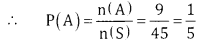(b) Let B: sum is less than 4
B = {(0, 1) (0, 2) (0, 3) (1, 2)} n(B) = 4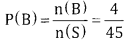(c) Let C: one number is 3 times the other
C = ((1,3) (2,6)(3,9)} ⇒ n(C)=3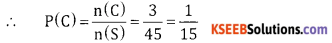error: Content is protected !!